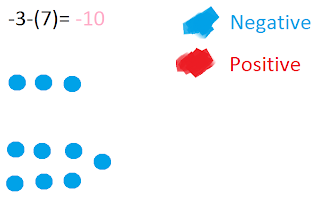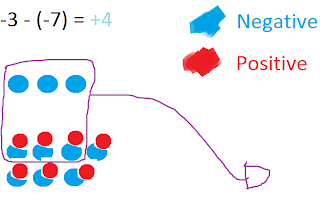## Monday, March 7, 2011

### Lizelle's Great Big Book Of Integers

Chapter 1 Grade 7 Integer Review:

First question:Question 2Question 4Question 5Question 6Question 7Question 8:Chapter 2: Multiplying Integers
(+2) x (+3)= (+2) x (-3)= (-2) x (+3)= (-2) x (-3)=
(+2) x (+3) =
(+2) x (-3) =
(-2) x (+3) =
(-2) x (-3) =

Chapter 3: Dividing Integers
(+2) x (+3)= (+2) x (-3)= (-2) x (+3)= (-2) x (-3)=
(+2) x (+3)= (+2) x (-3)= (-2) x (+3)= (-2) x (-3)=

Chapter 4

Order of Operations with Integers

Steps to solve (+5) x (-3) + (-6) ÷ (+3)=

1. Put square brackets around the operation that has to be done first (multiplication, division, addition or subtraction in order from left to right) and solve.